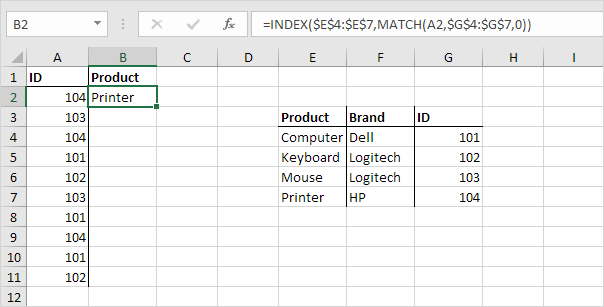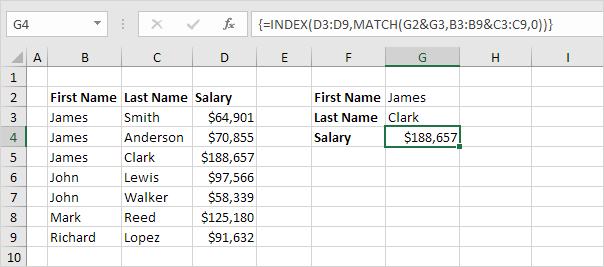# Index and Match

Use INDEX and MATCH in Excel and impress your boss. Instead of using VLOOKUP, use INDEX and MATCH. To perform advanced lookups, you’ll need INDEX and MATCH.

### Match

The MATCH function returns the position of a value in a given range. For example, the MATCH function below looks up the value 53 in the range B3:B9.Explanation: 53 (first argument) found at position 5 in the range B3:B9 (second argument). In this example, we use the MATCH function to return an exact match so we set the third argument to 0.

### Index

The INDEX function below returns a specific value in a one-dimensional range.Explanation: the INDEX function returns the 5th value (second argument) in the range E3:E9 (first argument).

### Index and Match

Replace the value 5 in the INDEX function (see previous example) with the MATCH function (see first example) to lookup the salary of ID 53.Explanation: the MATCH function returns position 5. The INDEX function needs position 5. It’s a perfect combination. If you like, you can also use the VLOOKUP function. It’s up to you. However, you’ll need INDEX and MATCH to perform advanced lookups, as we will see next.

### Two-way Lookup

The INDEX function can also return a specific value in a two-dimensional range. For example, use INDEX and MATCH in Excel to perform a two-way-lookup.### Case-sensitive Lookup

By default, the VLOOKUP function performs a case-insensitive lookup. However, you can use INDEX, MATCH and EXACT in Excel to perform a case-sensitive lookup.Note: the formula correctly looks up the salary of MIA Reed, not Mia Clark.

### Left Lookup

The VLOOKUP function only looks to the right. No worries, you can use INDEX and MATCH in Excel to perform a left lookup.Note: when we drag this formula down, the absolute references (\$E\$4:\$E\$7 and \$G\$4:\$G\$7) stay the same, while the relative reference (A2) changes to A3, A4, A5, etc.

### Two-column Lookup

Do you want to look up a value based on multiple criteria? Use INDEX and MATCH in Excel to perform a two-column lookup.Note: the array formula above looks up the salary of James Clark, not James Smith, not James Anderson.

### Closest Match

To find the closest match to a target value in a data column, use INDEX, MATCH, ABS and MIN in Excel.### Xlookup

If you have Excel 365 or Excel 2021, use XLOOKUP instead of INDEX and MATCH. The XLOOKUP function is easier to use and has some additional advantages.